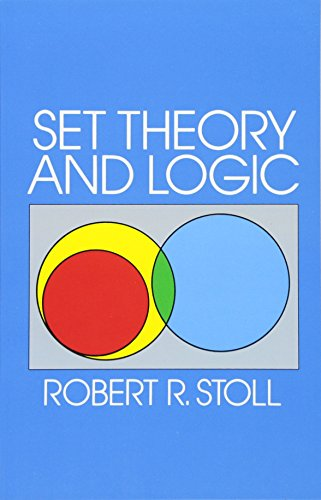# Set Theory and Logic (Dover Books on Mathematics)Type
Book
Authors
ISBN 10
0486638294
ISBN 13
9780486638294
Category
Unknown  [ Browse Items ]
Edition
Reprint
Publication Year
1979
Publisher
Pages
512
Abstract

Description
Set Theory and Logic is the result of a course of lectures for advanced undergraduates, developed at Oberlin College for the purpose of introducing students to the conceptual foundations of mathematics. Mathematics, specifically the real number system, is approached as a unity whose operations can be logically ordered through axioms. One of the most complex and essential of modern mathematical innovations, the theory of sets (crucial to quantum mechanics and other sciences), is introduced in a most careful concept manner, aiming for the maximum in clarity and stimulation for further study in set logic. Contents include: Sets and Relations — Cantor's concept of a set, etc.Natural Number Sequence — Zorn's Lemma, etc.Extension of Natural Numbers to Real NumbersLogic — the Statement and Predicate Calculus, etc.Informal Axiomatic MathematicsBoolean AlgebraInformal Axiomatic Set TheorySeveral Algebraic Theories — Rings, Integral Domains, Fields, etc.First-Order Theories — Metamathematics, etc.Symbolic logic does not figure significantly until the final chapter. The main theme of the book is mathematics as a system seen through the elaboration of real numbers; set theory and logic are seen s efficient tools in constructing axioms necessary to the system. Mathematics students at the undergraduate level, and those who seek a rigorous but not unnecessarily technical introduction to mathematical concepts, will welcome the return to print of this most lucid work. "Professor Stoll . . . has given us one of the best introductory texts we have seen." — Cosmos. "In the reviewer's opinion, this is an excellent book, and in addition to its use as a textbook (it contains a wealth of exercises and examples) can be recommended to all who wish an introduction to mathematical logic less technical than standard treatises (to which it can also serve as preliminary reading)." — Mathematical Reviews. - from Amzon
Biblio Notes

Number of Copies